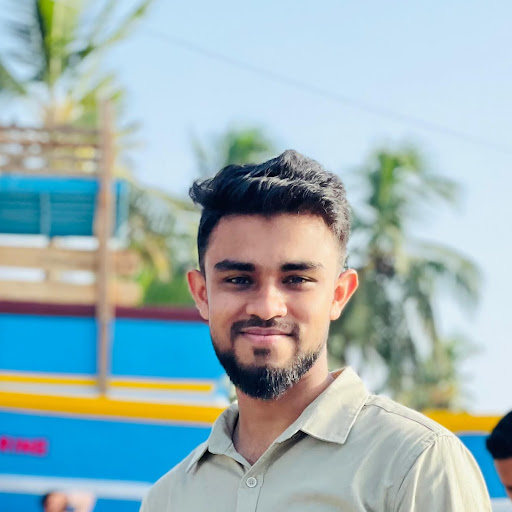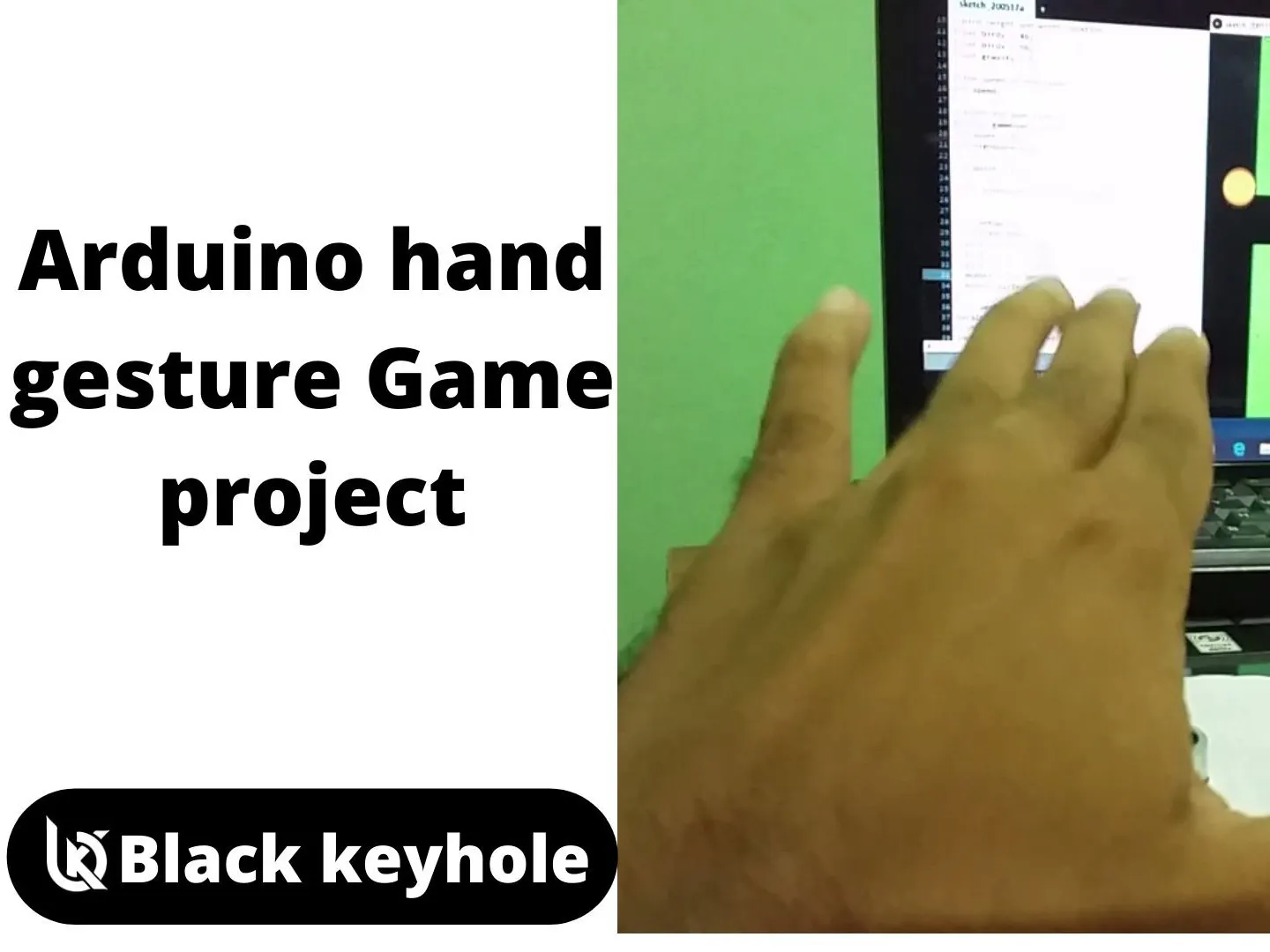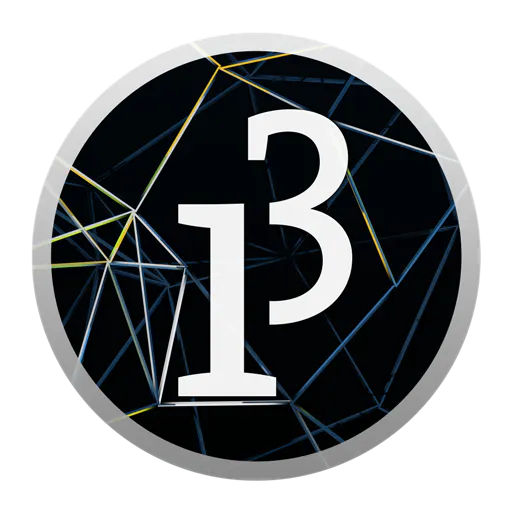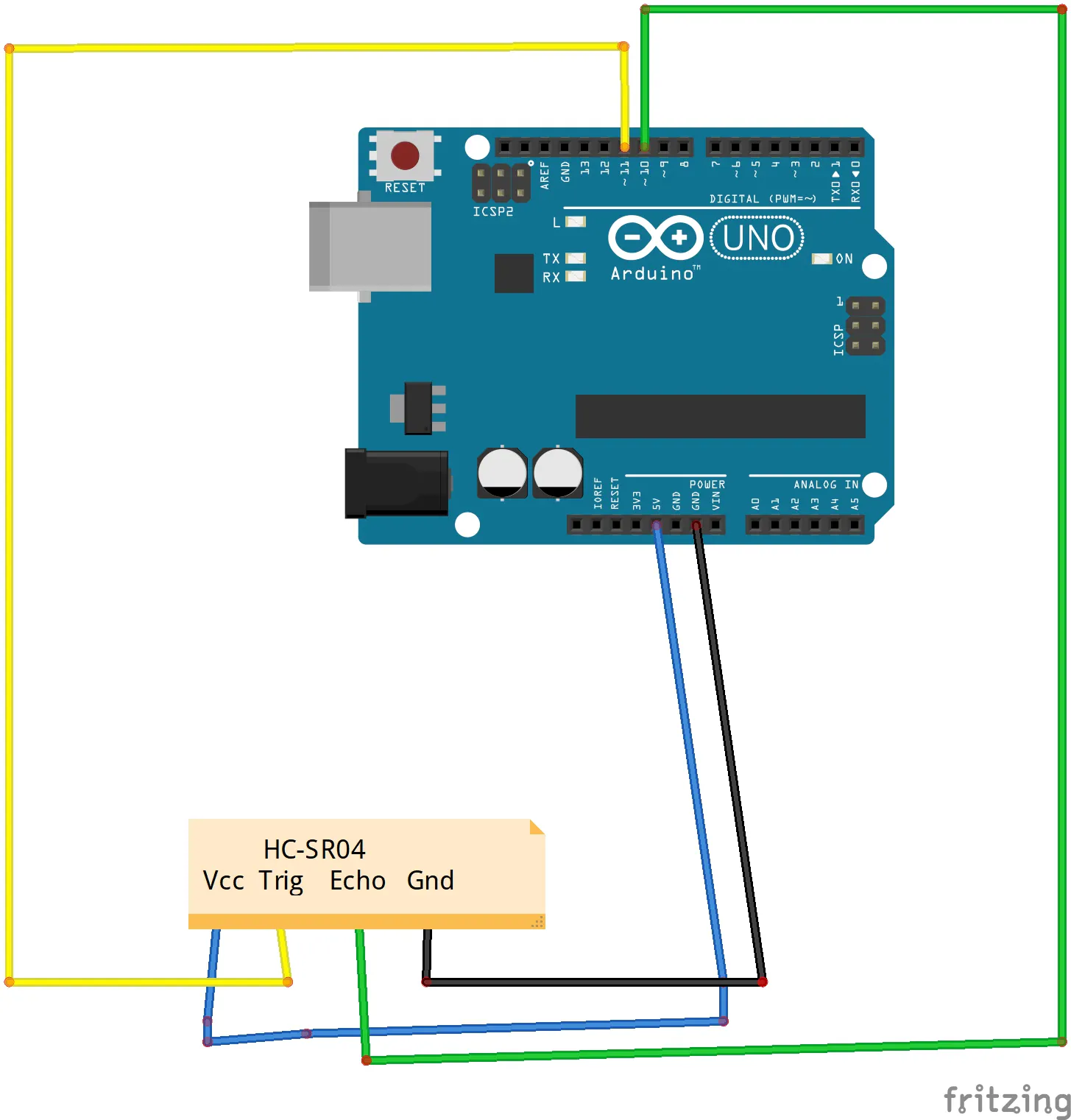Published

# Arduino hand gesture Game project

In this project, you can play the game using your hand gesture

BeginnerFull instructions provided94## Things used in this project

### Hardware componentsArduino UNO & Genuino UNO
×1UTSOURCE Electronic Parts
×1Ultrasonic Sensor - HC-SR04 (Generic)
×1

### Software apps and online servicesArduino IDEThe Processing Foundation Processing

## Schematics

### Cicuit## Code

### Arduino_Code

C/C++
```//for more projects visit www.blackkeyhole.com
const int trigPin=11;  //DECLARE TRIG PIN AT D11
int echoPin=10;         //DECLARE ECHO PIN AT D10
int safezone=10;
void setup()
{
pinMode(trigPin,OUTPUT);
pinMode(echoPin,INPUT);
Serial.begin(9600);
}
void loop()
{
long duration,cm;           //DECLARE VARIABLES TO STORE SENSOR O/P
digitalWrite(trigPin,LOW);  //MAKE THE TRIG PIN LOW
delayMicroseconds(2);       //WAIT FOR FEW MICROSECONDS
digitalWrite(trigPin,HIGH); //NOW SET THE TRIG PIN
delayMicroseconds(5);       //WAIT FOR FEW MICROSECONDS UNTIL THE TRIG PULSE IS SENT
digitalWrite(trigPin,LOW);  //MAKE THE TRIG PIN LOW AGAIN
duration=pulseIn(echoPin,HIGH); //MAKE ECHO PIN HIGH AND STORE THE BOUNCED PULSE IN VARIABLE DURATION
cm=microsecondsToCentimeters(duration);
long inch= cm/2.54;
Serial.println(cm);
}
long microsecondsToCentimeters(long microseconds) //SPEED OF SOUND IS 29 uSEC PER CM
{
return microseconds/29/2;  //THE PULSE TRAVELS FROM THE SENSOR AND AGAIN COMES BACK SO WE DIVIDE IT BY 2 TO TAKE ONLY HALF OF THE TOTAL DISTANCE
}
```

### Processing_code

C/C++
```import processing.serial.*;
int DistanceUltra;
int IncomingDistance;
Serial myPort;
String DataIn;
Pipe p1 = new Pipe();
Pipe p2 = new Pipe();
Pipe p3 = new Pipe();

//bird height and width location
float birdy = 46;
float birdx = 56;
float gravity = 5;

//the speed of the pipes
int speed;

//score and game state
boolean gameOver = false;
int score = 0;
int highscore = 0;

int point = 1;

color birdColor = color(255, 204, 0);

void setup(){
size(400,600);
p1.x = width + 50;
p2.x = width + 220;
p3.x = width + 370;
myPort = new Serial(this, "COM3", 9600); //change the com port which is suitable for your machine
myPort.bufferUntil(10);
}
void serialEvent (Serial myPort){
println(DataIn);
IncomingDistance = int(trim(DataIn));
println("incoming distance="+IncomingDistance);
if (IncomingDistance>1 && IncomingDistance<100 ) { DistanceUltra = IncomingDistance; //save the value only if its in the range 1 to 100 } }
}
}

void draw(){

background(0);
p1.pipe();
p2.pipe();
p3.pipe();

fill(birdColor);
ellipse(birdx, birdy, 55,55);
// birdy += gravity;
play();
success(p1);
success(p2);
success(p3);

if (IncomingDistance>10)
{
//birdy -= jumpForce;
birdy -= gravity;
}
else
{
birdy += gravity;
}

}

void play(){

if(gameOver == false)
{
speed = 2;
p1.x -= speed;
p2.x -= speed;
p3.x -= speed;

textSize(24);
fill(255,255,255);
text(score, width/2, 30);
}

if(gameOver == true)
{
speed = 0;
p1.x -= speed;
p2.x -= speed;
p3.x -= speed;

if( highscore < score)
{
highscore = score;
}

textSize(16);
fill(0, 102, 153);
textAlign(CENTER);
text("Click : Play Again", width/2, height/2);
text("Score: " + score, width/2, height/2 - 20);
text("High-Score: " + highscore, width/2, height/2 - 40);

if (mousePressed)
{
delay(900);
score = 0;
gameOver = false;
birdy = 100;
birdx = 56;
p1.x = width + 50;
p2.x = width + 220;
p3.x = width + 370;
p1.top = random(height/2);
p1.bottom = random(height/2);
p2.top = random(height/2);
p2.bottom = random(height/2);
p3.top = random(height/2);
p3.bottom = random(height/2);

}
}

}

void success(Pipe test){

if(birdy < test.top || birdy > height - test.bottom)
{
if(birdx > test.x && birdx < test.x + test.w)
{
gameOver = true;
}
}
}
class Pipe
{
float top = random(height/3 + 200);
float bottom = random(height/3 +200);

float x = width + 150;
float w = 70;
color pipeColor = color(0, 255, 0);

void pipe()
{
fill(pipeColor);
rect(x, 0, w, top);
rect(x, height-bottom, w, bottom);

if(x < -100)
{
score += point;
x = width;
top = random(height/2);
bottom = random(height/2);
}

}

}
```

## Credits

### Sharifdeen Ashshak

15 projects • 3 followers